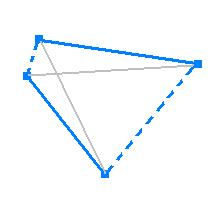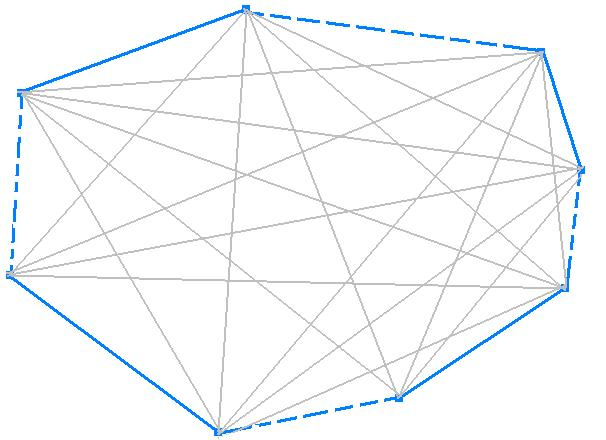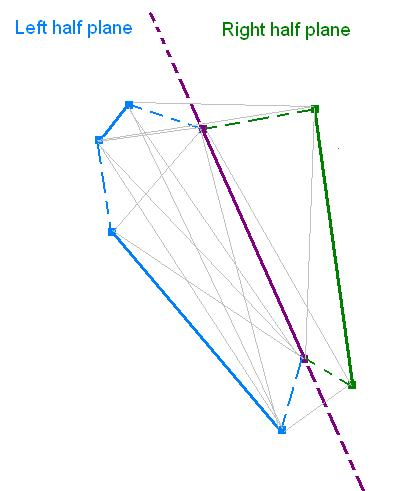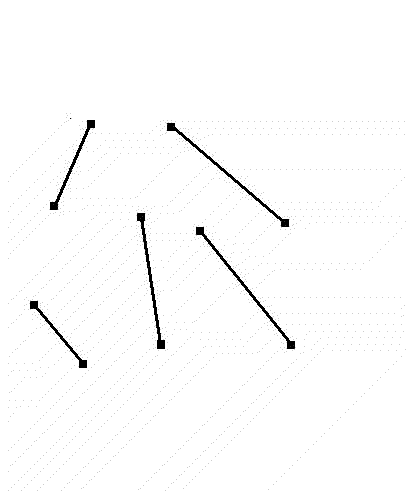Hamiltonian Visibility Graphs in Sets of Independent Segments

created by Joshua Auerbach

for Computational Geometry (Comp-507) Fall 2004

Introduction

This web site presents a proof and algorithms regarding the existence of simple Hamiltonian cycles in visibility graphs for a special cases of line segments: independent segments. The majority of the theory found within this page was from a paper by Joseph O'Rourke and Jennifer Rippel .

[ Introduction | Definitions | Theory | Algorithm | Applet | References ]

Definitions

• "convex hull"- the convex hull of a set of points S is the smallest convex polygon containing every point in S.
• "endpoint visibility graph" - the [endpoint] visibility graph, G=(V,E) of a set S of closed, disjoint line segments has a vertex v in V for every segment endpoint. Two vertices u and v are connected by an edge, if and only if the corresponding line segment uv is either in S or if the open segment uv does not intersect any segment from S [1,3]
• "hamiltonian cycle" - a cycle that visits each vertex of a graph exactly once
• "independent segments" - a set S of segments is independent if for each segment s in S the line containing s does not intersect any other segment in S 
• "integer lattice" - a regularly spaced array of points in a square array, i.e., points with coordinates (m,n,...), where m, n, ... are integers 
• "simple Hamiltonian cycle" - a planar Hamiltonian cycle that does not touch itself. It corresponds to a simple polygon 
• "unit lattice segments" - a set S of segments is a set of unit lattice segments if S is disjoint with endpoints on the integer lattice and each of unit length (so all segments are vertical or horizontal) 
• "visibility graph" - see endpoint visibility graph

Theory

Theorem: For any set, S, of at least 2 independent segments, there exists a Hamiltonian Cycle, C, on the visibility graph of S such that every segment lying on the convex hull of S is included in C.

Proof:

This theorem is proven by induction on the number of segments, n, in the set S.

Base Case:

If n = 2. Both segments must lie on the convex hull of S because they are independent, and therefore we just follow the convex hull of S and we get our Hamiltonian Cycle, C.Induction Hypothesis:

Assume the theorem holds for n = 3,4,....k - 1 segments

Consider a set S of n=k independent segments

From the n independent segments we choose a segment s, not on the convex hull of S. If there is no such s, than all segments are on the convex hull and once again we may just follow the convex hull of S and we get our Hamiltonian Cycle, C.Otherwise arbitrarily choose one such s and divide the set S into two subsets:

L = the segment s, and all segments to the left of s

R = the segment s, and all segments to the right of s.

Since s is not on the convex hull of S it must have segments on either side of it, so the sets L and R both contain at least 2 segments each. Assume that L contains 1<a<k segments, so R would contain k-a+1 segments, where 1<k-a+1<k.

So we can apply the induction hypothesis on both and L and R to get that both subsets contain the desired Hamiltonian Cycles. Furthermore both of these cycles contain both vertices of the segment s, so we simply remove s from each cycle and paste the remainder of our cycles together to get the desired cycle for our set S. QED[ Introduction | Definitions | Theory | Algorithm | Applet | References ]

Algorithm

A recursive algorithm for finding a Hamiltonian Cycle on the endpoint visibility graph of a set of independent segments follows directly from this inductive proof.[ Introduction | Definitions | Theory | Algorithm | Applet | References ]

Java Applet

This applet allows you to input a set of (up to 500) independent line segments and will find a Hamiltonian Cycle on the visibility graph of these segments using the recursive algorithm presented above. There are options to show the visibility graph of the set of segments and to show the convex hull of the set of segments. (View source code here)

[ Introduction | Definitions | Theory | Algorithm | Applet | References ]

References

 O'Rourke, J. and Rippel J. Two Segment Classes with Hamiltonian Visibility Graphs. Computational Geometry Theory and Applications (1994) 209-218

 Integer Lattice. Mathworld. http://mathworld.wolfram.com/IntegerLattice.html

 Hoffman, M. and Toth, C. Segment Endpoint Visibility Graphs. http://www.ti.inf.ethz.ch/ew/workshops/uraw02/abs-2042/abs-2042.html

[ Introduction | Definitions | Theory | Algorithm | Applet | References ]